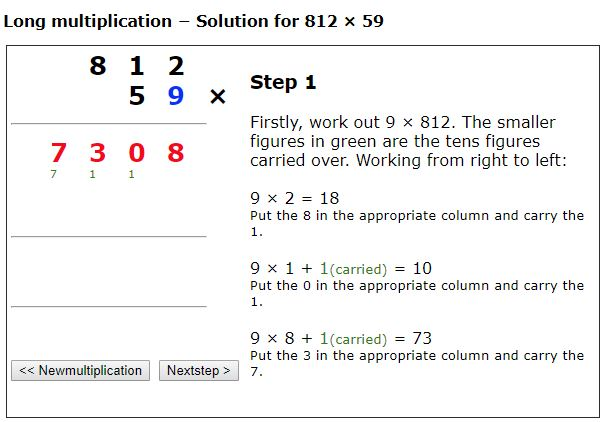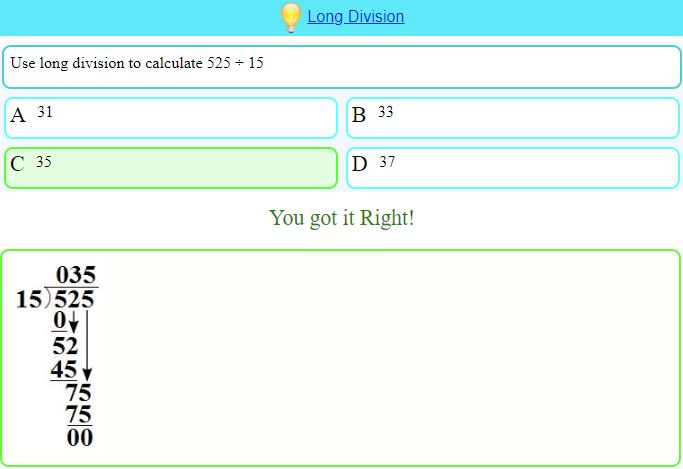# Long Division & Multiplication – Formal Methods

The UK National Curriculum now specifies that:
Pupils should be taught to:

Year 6 (UK KS2 age 10-11)

• multiply multi-digit numbers up to 4 digits by a two-digit whole number using the formal written method of long multiplication
• divide numbers up to 4 digits by a two-digit whole number using the formal written method of long division, and interpret remainders as whole number remainders, fractions, or by rounding, as appropriate for the context
• divide numbers up to 4 digits by a two-digit number using the formal written method of short division where appropriate, interpreting remainders according to the context

Appendix 1 of the KS2 document includes the examples below and states that “the examples of formal written methods for all four operations illustrate the range of methods that could be taught. It is not intended to be an exhaustive list, nor is it intended to show progression in formal written methods. For example, the exact position of intermediate calculations (superscript and subscript digits) will vary depending on the method and format used. For multiplication, some pupils may include an addition symbol when adding partial products. For division, some pupils may include a subtraction symbol when subtracting multiples of the divisor.

And at KS3 (UK age 11-14) we are reminded that students should be able to “use the four operations, including formal written methods, applied to integers, decimals, proper and improper fractions, and mixed numbers, all both positive and negative”.From MathsOnline, comes a clear step by step demonstration of long multiplication, (uses html). Each step is clearly explained. Long Division is also very clearly illustrated and for your older students we can extend to Algebraic Long Division. Refreshing the page generates a new example each time.Mathisfun explains long division very clearly and additionally offers questions and worksheets. Similar resources are available for Long Multiplication and for

For examples, exercises and problems try the ever reliable CIMT’s GCSE chapter 6 on the Number System – see Section 6.4.

## 3 comments on “Long Division & Multiplication – Formal Methods”

1.creatingmathsheroes

I will have to check and make sure this isn’t in the Australian curriculum – yes students need to be able to do multiplying and dividing but there is certainly more than one way to do it!

•Colleen Young

There is indeed more than one way – but for UK now certainly at KS2 & 3 these methods are specified – relatively recent here.

The site mentioned here, sums.co.uk has many other goodies, including for multiplication and division – worth exploring!